# Test Prep Plan - Take a practice test

Take this practice test to check your existing knowledge of the course material. We'll review your answers and create a Test Prep Plan for you based on your results.
How Test Prep Plans work
1
2Based on your results, we'll create a customized Test Prep Plan just for you!
3Study smarter
Study more effectively: skip concepts you already know and focus on what you still need to learn.

# GACE Middle Grades Math: Triangles Chapter Exam

Exam Instructions:

Choose your answers to the questions and click 'Next' to see the next set of questions. You can skip questions if you would like and come back to them later with the yellow "Go To First Skipped Question" button. When you have completed the practice exam, a green submit button will appear. Click it to see your results. Good luck!

### Page 1

#### Question 1 1. What type of triangle is pictured?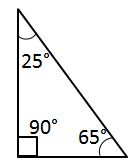#### Question 2 2. What type of triangle is pictured?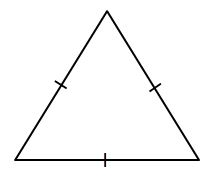#### Question 5 5. Mary claims that the triangles below are similar by AA. Do you agree or disagree with Mary?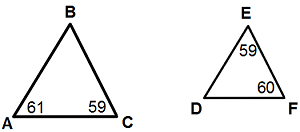### Page 2

#### Question 6 6. Given the triangle PQE, calculate the measure of Angle Q.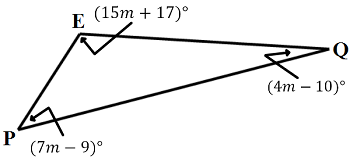#### Question 7 7. In triangle GBN, Angle G is seven more than three times a number, Angle B is five more than four times the number, and Angle N is twelve less than five times the number. Solve for the measure of Angle G.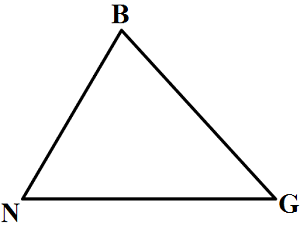#### Question 8 8. Determine if triangle VEZ is similar to triangle MAG.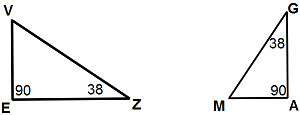#### Question 10 10. In the pictured triangle, what is side B, relative to angle x?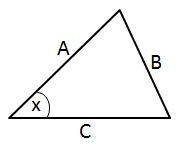### Page 3

#### Question 11 11. If angle a is 80 degrees, what is the measure of angle d?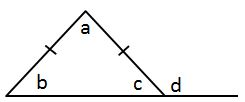#### Question 12 12. What type of line segment is represented by the dotted line in the pictured triangle?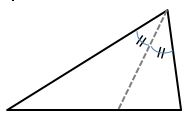#### Question 14 14. What type of triangle is pictured?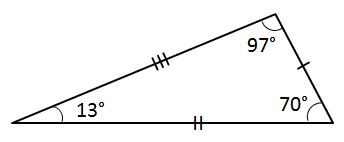#### Question 15 15. What type of line segment is represented by the dotted line in the pictured triangle?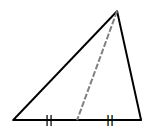### Page 4

#### Question 16 16. Conclude whether triangle BEN is similar to triangle MAT.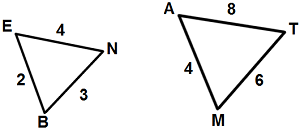#### Question 17 17. In the pictured triangle, what is the centroid?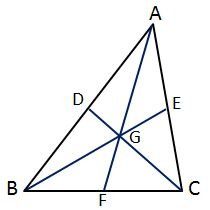#### Question 18 18. In the pictured triangle, which of the following lines is an altitude?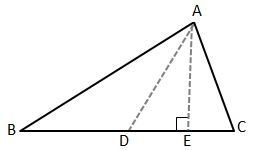#### Question 19 19. In the pictured triangle, what is side A, relative to angle x?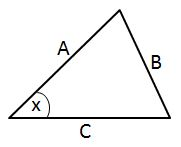### Page 5

#### Question 21 21. What type of triangle is pictured?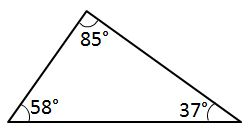#### Question 23 23. Which statement is true about the pictured triangle?#### Question 24 24. Given the triangle ABC, solve for the value of y.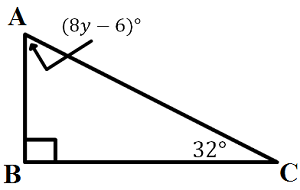### Page 6

#### Question 26 26. In the pictured triangle, identify the altitude(s).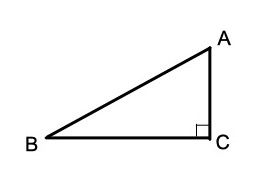#### Question 28 28. If angle a is 68 degrees and angle b is 37 degrees, what is the measure of angle c?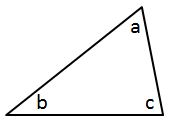#### Question 29 29. What type of triangle is pictured?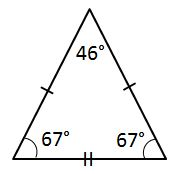#### Question 30 30. In the figure below, Angle 7 = 15x and Angle 10 = 9x + 30. Determine the measure of Angle 10.#### GACE Middle Grades Math: Triangles Chapter Exam Instructions

Choose your answers to the questions and click 'Next' to see the next set of questions. You can skip questions if you would like and come back to them later with the yellow "Go To First Skipped Question" button. When you have completed the practice exam, a green submit button will appear. Click it to see your results. Good luck!

Support